TitleCollege Algebra
Answer/Discussion to Practice Problems
Tutorial 12: Complex Numbers

WTAMU > Virtual Math Lab > College Algebra > Tutorial 12: Complex NumbersAnswer/Discussion to 1a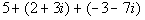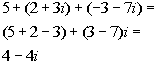*Add the real num. together and the imaginary num. together *Complex num. in stand. formAnswer/Discussion to 1b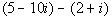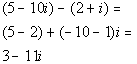*Subtract the real num. together and the imaginary num. together *Complex num. in stand. formAnswer/Discussion to 1c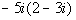Step 1:  Multiply the complex numbers in the same manner as polynomials AND Step 2:  Simplify the expression.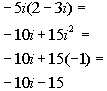*Use dist. prop. to multiply *i squared = -1

 Step 3:  Write the final answer in standard form.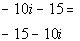*Complex num. in stand. formAnswer/Discussion to 1dStep 1:  Multiply the complex numbers in the same manner as polynomials AND Step 2:  Simplify the expression.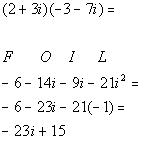*Use FOIL method to multiply *Combine imaginary numbers *i squared = -1

 Step 3:  Write the final answer in standard form.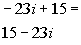*Complex num. in stand. formAnswer/Discussion to 1eStep 1:  Find the conjugate of the denominator.

 In general the conjugate of a + bi is a - bi and vice versa.  So what would the conjugate of our denominator be?  It looks like the conjugate is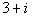.

 Step 2:  Multiply the numerator and the denominator of the fraction by the conjugate found in Step 1 AND Step 3:  Simplify the expression.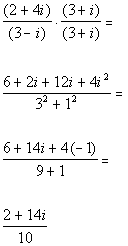*Multiply top and bottom by conj. of den.     *Step 4:  Write the final answer in standard form.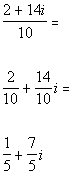*Divide each term of num. by 10     *Complex num. in stand. formAnswer/Discussion to 1f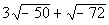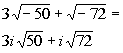*Square root of a negative is i

 Step 2:  Perform the indicated operation AND Step 3:  Write the final answer in standard form.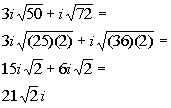*Rewrite 50 as (25)(2) and 72 as (36)(2) *The square root of 4 is 2 and the square root of 36 is 6 *Add like radicals: 15i + 6i = 21i *Complex num. in stand. form (note real num. part is 0)Answer/Discussion to 1g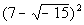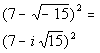*Square root of a negative is i

 Step 2:  Perform the indicated operation AND Step 3:  Write the final answer in standard form.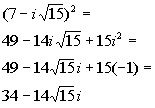*i squared = -1 *Complex num. in stand. formAnswer/Discussion to 1h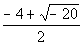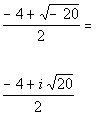*Square root of a negative is i

 Step 2:  Perform the indicated operation AND Step 3:  Write the final answer in standard form.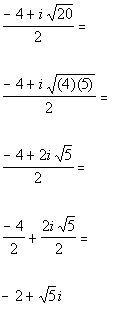*Rewrite 20 as (4)(5)      *The square root of 4 is 2     *Divide each term of num. by 2     *Complex num. in stand. formAnswer/Discussion to 1i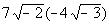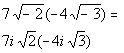*Square root of a negative is i

 Step 2: Perform the indicated operation AND Step 3:  Write the final answer in standard form.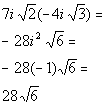*i squared = -1 *Complex num. in stand. form (note that the imaginary part is 0)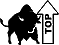WTAMU > Virtual Math Lab > College Algebra > Tutorial 12: Complex Numbers

Last revised on Dec. 15, 2009 by Kim Seward.
All contents copyright (C) 2002 - 2010, WTAMU and Kim Seward. All rights reserved.